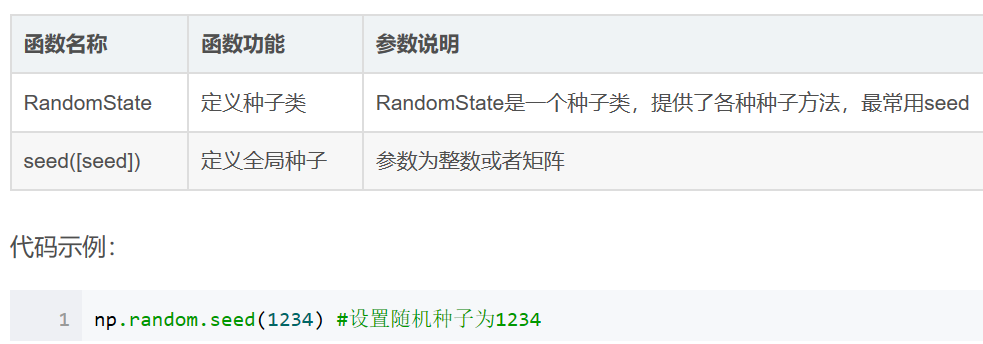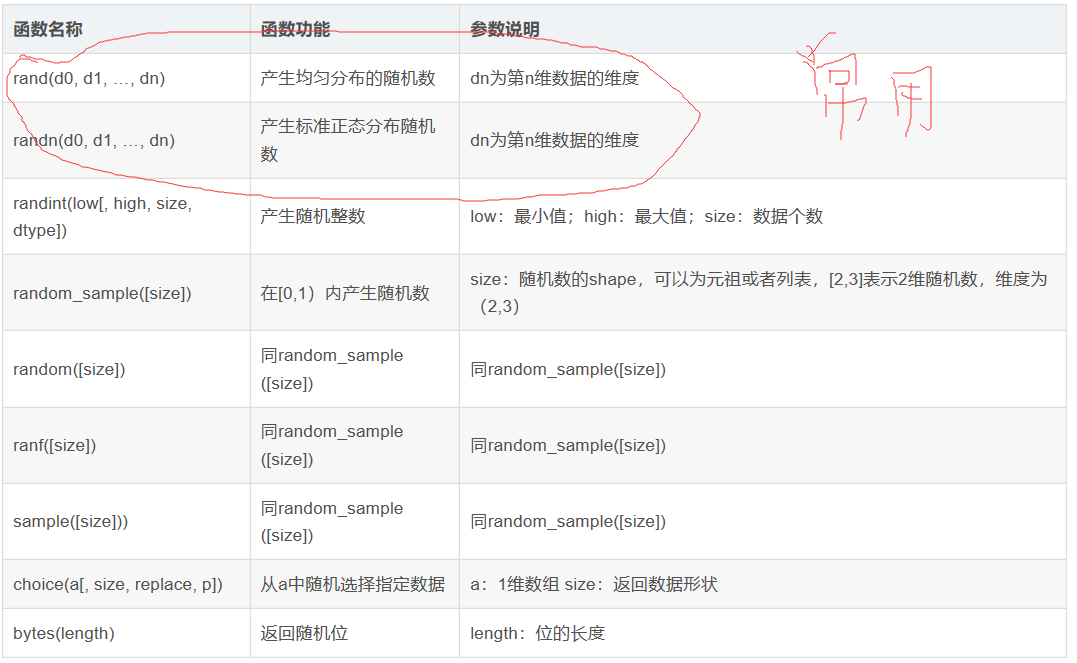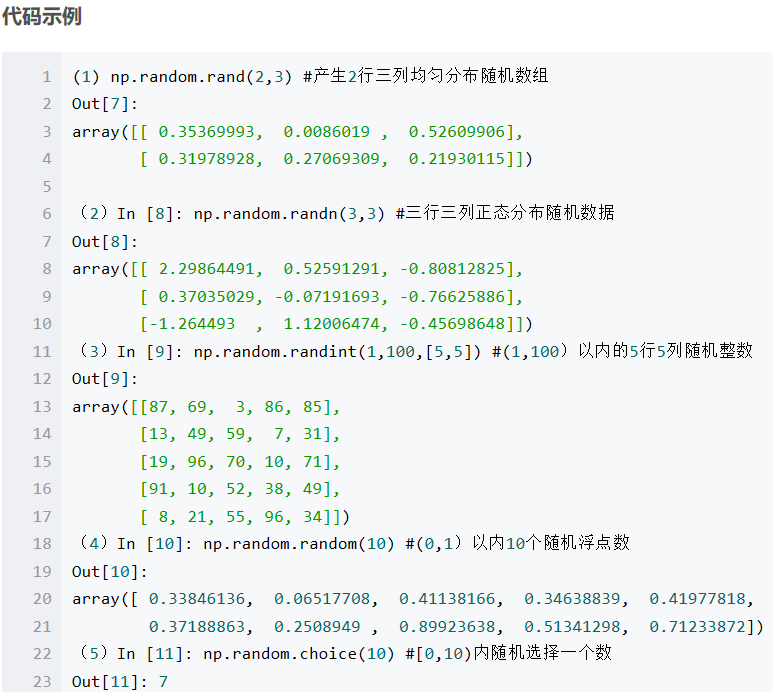• 如果你对在Python生成随机数与random模块中最常用的几个函数的关系与不懂之处，下面的文章就是对Python生成随机数与random模块中最常用的几个函数的关系，希望你会有所收获，以下就是这篇文章的介绍。 random....
如果你对在Python生成随机数与random模块中最常用的几个函数的关系与不懂之处，下面的文章就是对Python生成随机数与random模块中最常用的几个函数的关系，希望你会有所收获，以下就是这篇文章的介绍。

random.random()用于生成

用于生成一个指定范围内的随机符点数，两个参数其中一个是上限，一个是下限。如果a > b，则生成随机数

1

n: a <= n <=  b。如果 a <b， 则 b <= n <= a。

1

2

3

4

5

6

print random.uniform(10, 20)  

print random.uniform(20, 10)  

#---- 

#18.7356606526  

#12.5798298022  

random.randint

用于生成一个指定范围内的整数。其中参数a是下限，参数b是上限，Python生成随机数

1

2

3

print random.randint(12, 20) #生成的随机数n: 12 <= n <= 20 

print random.randint(20, 20) #结果永远是20 

#print random.randint(20, 10) #该语句是错误的。 

下限必须小于上限。

random.randrange

从指定范围内，按指定基数递增的集合中 ，这篇文章就是对python生成随机数的应用程序的部分介绍。

随机整数：>>> import random
>>> random.randint(0,99)
21

随机选取0到100间的偶数：>>> import random
>>> random.randrange(0, 101, 2)
42

随机浮点数：>>> import random
>>> random.random()
0.85415370477785668
>>> random.uniform(1, 10)
5.4221167969800881

随机字符：>>> import random
>>> random.choice('abcdefg&#%^*f')
'd'

多个字符中选取特定数量的字符：>>> import random
random.sample('abcdefghij',3)
['a', 'd', 'b']

多个字符中选取特定数量的字符组成新字符串：>>> import random
>>> import string
>>> string.join(random.sample(['a','b','c','d','e','f','g','h','i','j'], 3)).r
eplace(" ","")
'fih'

随机选取字符串：>>> import random
>>> random.choice ( ['apple', 'pear', 'peach', 'orange', 'lemon'] )
'lemon'

洗牌：>>> import random
>>> items = [1, 2, 3, 4, 5, 6]
>>> random.shuffle(items)
>>> items
[3, 2, 5, 6, 4, 1]扫一扫 关注微信公众号！号主 专注于搜索和推荐系统，尝试使用算法去更好的服务于用户，包括但不局限于机器学习，深度学习，强化学习，自然语言理解，知识图谱，还不定时分享技术，资料，思考等文章！

【技术服务】，详情点击查看：https://mp.weixin.qq.com/s/PtX9ukKRBmazAWARprGIAg展开全文random 函数
• ## Python中产生随机数

万次阅读 多人点赞 2019-07-09 18:42:27
Python产生随机数： 一.Python自带的random库 1.参生n--m范围内的一个随机数: random.randint(n,m) 2.产生0到1之间的浮点数: random.random() 3.产生n---m之间的浮点数: random.uniform(1.1,5.4) 4.产生从n...
Python产生随机数：

一.Python自带的random库

1.参生n--m范围内的一个随机数:    random.randint(n,m)

2.产生0到1之间的浮点数:  random.random()

3.产生n---m之间的浮点数:  random.uniform(1.1,5.4)

4.产生从n---m间隔为k的整数: random.randrange(n,m,k)

5.从序列中随机选取一个元素:  random.choice([1, 2, 3, 4, 5, 6, 7, 8, 9, 0])

6.在一些特殊的情况下可能对序列进行一次打乱操作: random.shuffle([1,3,5,6,7])

import random

# 产生 1 到 10 的一个整数型随机数
print( random.randint(1,10) )
# 产生 0 到 1 之间的随机浮点数
print( random.random() )
# 产生  1.1 到 5.4 之间的随机浮点数，区间可以不是整数
print( random.uniform(1.1,5.4) )
# 从序列中随机选取一个元素
print( random.choice([1, 2, 3, 4, 5, 6, 7, 8, 9, 0]) )
# 生成从1到100的间隔为2的随机整数
print( random.randrange(1,100,2) )
# 将序列a中的元素顺序打乱
a=[1,3,5,6,7]
random.shuffle([1,3,5,6,7])
print(a)

二.numpy库

1.产生N维的均匀分布的随机数:  np.random.rand(d1,d2,d3,...,dn)

2.产生n维的正态分布的随机数:   np.random.randn(d1,d2,d3,...,dn)

3.产生n--m之间的k个整数：np.random.randint(n,m,k)

4.产生n个0--1之间的随机数: np.random.random(10)

5.从序列中选择数据: np.random.choice([2,5,7,8,9,11,3])

6.把序列中的数据打乱：np.random.shuffle(item)

import numpy as np

#产生n维的均匀分布的随机数
print(np.random.rand(5,5,5))

#产生n维的正态分布的随机数
print(np.random.randn(5,5,5))

#产生n--m之间的k个整数
print(np.random.randint(1,50,5))

#产生n个0--1之间的随机数
print(np.random.random(10))

#从序列中选择数据
print(np.random.choice([2,5,7,8,9,11,3]))

#把序列中的数据打乱
#np.random.shuffle(item) 不会参数返回值，改变的话是在原列表中修改的
item = [2,5,7,8,9,11,3]
np.random.shuffle(item)
print(item)


展开全文• ## Python 产生随机数

万次阅读 2018-08-05 16:28:52
在数据分析中，数据的获取是第一步，numpy.random 模块提供了非常全的自动产生数据API，是学习数据分析的第一步。 总体来说，numpy.random模块...4. 生成器：种随机数种子，根据同一种子产生的随机数是相同的 以下是...


在数据分析中，数据的获取是第一步，numpy.random 模块提供了非常全的自动产生数据API，是学习数据分析的第一步。
总体来说，numpy.random模块分为四个部分，对应四种功能：
1. 简单随机数： 产生简单的随机数据，可以是任何维度
2. 排列：将所给对象随机排列
3. 分布：产生指定分布的数据，如高斯分布等
4. 生成器：种随机数种子，根据同一种子产生的随机数是相同的
以下是详细内容以及代码实例：（以下代码默认已导入numpy：import numpy as np ）

1. 生成器

电脑产生随机数需要明白以下几点：
（1）随机数是由随机种子根据一定的计算方法计算出来的数值。所以，只要计算方法一定，随机种子一定，那么产生的随机数就不会变。
（2）只要用户不设置随机种子，那么在默认情况下随机种子来自系统时钟（即定时/计数器的值）
（3）随机数产生的算法与系统有关，Windows和Linux是不同的，也就是说，即便是随机种子一样，不同系统产生的随机数也不一样。
numpy.random 设置种子的方法有：np.random.RandomState(42)

2、简单随机数例：

rng = np.random.RandomState(42) # 生成随机数时可指定一种子，目的是为了生成相同的随机数，实现代码的复现。

X = rng.randn(100, 2)

参考自：https://blog.csdn.net/jinxiaonian11/article/details/53143141

具体解读：

1、np.random.rand()

给定一个形状创建数组，并在数组中加入[0,1]之间均匀分布的随机样本。

numpy.random.rand(d0, d1, ..., dn)，产生d0 - d1 - ... - dn形状的在[0,1)上均匀分布的float型数。

2、np.random.randn()

以给定的形状创建一个数组，数组元素符合标准正态分布N(0,1)。

若要获得一般正态分布N(u,sigma2),可用 sigma*np.random.randn+u

numpy.random.randn（d0,d1,...,dn),产生d0 - d1 - ... - dn形状的标准正态分布的float型数。

3.numpy.random.randint()
用法是：numpy.random.randint(low,high=None,size=None,dtype)
生成在半开半闭区间[low,high)上离散均匀分布的整数值;若high=None，则取值区间变为[0,low)

numpy.random.randint(low, high=None, size=None, dtype='l')，产生随机整数

4.numpy.random.random_integers()
用法是： numpy.random.random_integers(low,high=None,size=None)
生成闭区间[low,high]上离散均匀分布的整数值;若high=None，则取值区间变为[1,low]

此外，若要将【a,b】区间分成N等分，也可以用此函数实现

numpy.random.random_integers(low, high=None, size=None)，在闭区间上产生随机整数；

a+(b-a)*(numpy.random.random_integers(N)-1)/(N-1)

5.numpy.random_sample()
用法是： numpy.random.random_sample(size=None)
以给定形状返回[0,1)之间的随机浮点数

6.numpy.random.choice()
用法是： numpy.random.choice(a,size=None,replace=True,p=None)
若a为数组，则从a中选取元素；若a为单个int类型数，则选取range(a)中的数
replace是bool类型，为True，则选取的元素会出现重复；反之不会出现重复
p为数组，里面存放选到每个数的可能性，即概率

7、函数原型：  numpy.random.uniform(low,high,size)

功能：从一个均匀分布[low,high)中随机采样，注意定义域是左闭右开，即包含low，不包含high.

参数介绍:

low: 采样下界，float类型，默认值为0；
high: 采样上界，float类型，默认值为1；
size: 输出样本数目，为int或元组(tuple)类型，例如，size=(m,n,k), 则输出m*n*k个样本，缺省时输出1个值。

返回值：ndarray类型，其形状和参数size中描述一致。

参考：https://blog.csdn.net/u013920434/article/details/52507173


展开全文• ## 用python生成随机数的几种方法

万次阅读 多人点赞 2018-07-13 20:32:27
今天学习了用python生成仿真数据的一些基本方法和技巧，写成博客和大家分享一下。 本篇博客主要讲解如何从给定参数的的正态分布/均匀分布中生成随机数以及如何以给定概率从数字列表抽取某数字或从区间列表的某一...
  今天学习了用python生成仿真数据的一些基本方法和技巧，写成博客和大家分享一下。      本篇博客主要讲解如何从给定参数的的正态分布/均匀分布中生成随机数以及如何以给定概率从数字列表抽取某数字或从区间列表的某一区间内生成随机数，按照内容将博客分为3部分，并附上代码。1 从给定参数的正态分布中生成随机数  当考虑从正态分布中生成随机数时，应当首先知道正态分布的均值和方差（标准差），有了这些，就可以调用python中现有的模块和函数来生成随机数了。这里调用了Numpy模块中的random.normal函数，由于逻辑非参简单，所有直接贴上代码如下：import numpy as np

# 定义从正态分布中获取随机数的函数
def get_normal_random_number(loc, scale):
"""
:param loc: 正态分布的均值
:param scale: 正态分布的标准差
:return:从正态分布中产生的随机数
"""
# 正态分布中的随机数生成
number = np.random.normal(loc=loc, scale=scale)
# 返回值
return number

# 主模块
if __name__ == "__main__":
# 函数调用
n = get_normal_random_number(loc=2, scale=2)
# 打印结果
print(n)
# 结果:3.2751924434630582 从给定参数的均匀分布中获取随机数的函数  考虑从均匀分布中获取随机数的时候，要事先知道均匀分布的下界和上界，然后调用Numpy模块的random.uniform函数生成随机数。import numpy as np

# 定义从均匀分布中获取随机数的函数
def get_uniform_random_number(low, high):
"""
:param low: 均匀分布的下界
:param high: 均匀分布的上界
:return: 从均匀分布中产生的随机数
"""
# 均匀分布的随机数生成
number = np.random.uniform(low, high)
# 返回值
return number

# 主模块
if __name__ == "__main__":
# 函数调用
n = get_uniform_random_number(low=2, high=4)
# 打印结果
print(n)
# 结果:2.44624171401531143 按照指定概率生成随机数  有时候我们需要按照指定的概率生成随机数，比如已知盒子中每种颜色的球的比例，猜测下一次取出的球的颜色。在这里介绍的问题和上面的例子相似，要求给定一个概率列表，从列表对应的数字列表或区间列表中生成随机数，分两部分讨论。3.1 按照指定概率从数字列表中随机抽取数字  假设给定一个数字列表和一个与之对应的概率列表，两个列表对应位置的元素组成的元组即表示该数字在数字列表中以多大的概率出现，那么如何根据这些已知条件从数字列表中按概率抽取随机数呢？在这里我们考虑用均匀分布来模拟概率，代码如下：import numpy as np
import random

# 定义从均匀分布中获取随机数的函数
def get_uniform_random_number(low, high):
"""
:param low: 均匀分布的下界
:param high: 均匀分布的上界
:return: 从均匀分布中产生的随机数
"""
# 均匀分布的随机数生成
number = np.random.uniform(low, high)
# 返回值
return number

# 定义从一个数字列表中以一定的概率取出对应区间中数字的函数
def get_number_by_pro(number_list, pro_list):
"""
:param number_list:数字列表
:param pro_list:数字对应的概率列表
:return:按概率从数字列表中抽取的数字
"""
# 用均匀分布中的样本值来模拟概率
x = random.uniform(0, 1)
# 累积概率
cum_pro = 0.0
# 将可迭代对象打包成元组列表
for number, number_pro in zip(number_list, pro_list):
cum_pro += number_pro
if x < cum_pro:
# 返回值
return number

# 主模块
if __name__ == "__main__":
# 数字列表
num_list = [1, 2, 3, 4, 5]
# 对应的概率列表
pr_list = [0.1, 0.3, 0.1, 0.4, 0.1]
# 函数调用
n = get_number_by_pro(number_list=num_list, pro_list=pr_list)
# 打印结果
print(n)
# 结果:13.2 按照指定概率从区间列表中的某个区间内生成随机数  给定一个区间列表和一个与之对应的概率列表，两个列表相应位置的元素组成的元组即表示某数字出现在某区间内的概率是多少，已知这些，我们如何生成随机数呢？这里我们通过两次使用均匀分布达到目的，代码如下：import numpy as np
import random

# 定义从均匀分布中获取随机数的函数
def get_uniform_random_number(low, high):
"""
:param low: 均匀分布的下界
:param high: 均匀分布的上界
:return: 从均匀分布中产生的随机数
"""
# 均匀分布的随机数生成
number = np.random.uniform(low, high)
# 返回值
return number

# 定义从一个数字列表中以一定的概率取出对应区间中数字的函数
def get_number_by_pro(number_list, pro_list):
"""
:param number_list:数字列表
:param pro_list:数字对应的概率列表
:return:按概率从数字列表中抽取的数字
"""
# 用均匀分布中的样本值来模拟概率
x = random.uniform(0, 1)
# 累积概率
cum_pro = 0.0
# 将可迭代对象打包成元组列表
for number, number_pro in zip(number_list, pro_list):
cum_pro += number_pro
if x < cum_pro:
# 从区间[number. number - 1]上随机抽取一个值
num = get_uniform_random_number(number, number - 1)
# 返回值
return num

# 主模块
if __name__ == "__main__":
# 数字列表
num_list = [1, 2, 3, 4, 5]
# 对应的概率列表
pr_list = [0.1, 0.3, 0.1, 0.4, 0.1]
# 函数调用
n = get_number_by_pro(number_list=num_list, pro_list=pr_list)
# 打印结果
print(n)
# 结果:3.49683787011193欢迎交流！    QQ：3408649893
展开全文• ## python 生成随机数的三种方法

万次阅读 多人点赞 2018-09-28 18:05:35
1. 使用 random 包生成随机数 可以生成 均匀分布， 高斯分布，（包括正态分布） 指数分布，（与泊松分布有区别：泊松分布表示一段时间发生多少次，而指数分布表示两次发生的时间间隔） 贝塔分布， 韦布尔分布的...random numpy scipy
• 随机种子 random.seed(num) num为空时为真随机，num为一个固定数时是固定的随机 普通随机 import random x = random.random() print x 固定范围整数随机-->半开区间 [low, high)。...np.rando
• 随机数组是非常有用的一种数组形式，特别是在测试中。 最直接的方式（numpy.random） 用numpy.random模块可方便生成随机数组，import numpy as np。 np.random.rand：生成[0.0, 1.0)之间的随机浮点数 ...
• ## Python中随机数的生成

万次阅读 多人点赞 2018-06-05 16:50:42
Python中可以用于随机数生成的有两种主要途径，一是random模块，另一个是numpy库中random函数。 OUTLINE random模块 numpy中的random函数 总结 random模块 random模块中将近有7个函数都是可以用来生成...
• 列表中有随机产生的10个数import randomL=[random.randint(0,10) for _ in range(10) ]random.randint(0,n)表示随机数的范围为：0到nfor _ in range(n)表示产生n随机数pytho
• python生成随机数、随机字符、随机字符串 本文是基于Windows 10系统环境，实现python生成随机数、随机字符、随机字符串： Windows 10 PyCharm 2018.3.5 for Windows (exe) python 3.6.8 Windows x86 ...
• 1.在某个范围生成x个随机数使用 numpy包 实现>>> import numpy as np >>> np.random.randint(1, 100, 10) array([23, 55, 7, 75, 22, 83, 35, 6, 24, 33])上述在0和100之间生成了10个随机数。2.生成无重复的随机数...
• 最直接的方式：用numpy.random模块来生成随机数组 1、np.random.rand 用于生成[0.0, 1.0)之间的随机浮点数， 当没有参数时，返回一个随机浮点数，当有一个参数时，返回该参数长度大小的一维随机浮点数数组，参数...
• Python中用于生成随机数的模块是random，在使用前需要import。 random.random():生成一个0-1之间的随机浮点数 random.randint(a,b):生成[a,b]之间的整数,包含a,b random.uniform(a,b):生成[a,b]之间的浮点数 ...
• 因为要做数值仿真实验，用到了随机生成模块random 因此，将相关用法列出如下： #todo 产生随机数的模块 import random random.uniform(3,4) # 3到4之间的均匀分布 random.gauss(5,1) # 以5为均值，1为方差的高斯...
• python中用于生成随机数的模块是random,在使用前需要import random.random: random.random():生成一个0-1之间的随机浮点数.例: import random print random.random() # 0.87594424128 random.uniform ...
• python语言随机生成一万个一到一百万的整数，并且保存十进制和二进制文件，最后采用快速排序排序#!D:/workplace/python # -*- coding: utf-8 -*- # @File : homework1.py # @Author: WangYe # @Date : 2018/3/20 #...快速排序
• 如果你对在Python生成随机数与random模块中最常用的几个函数的关系与不懂之处，下面的文章就是对Python生成随机数与random模块中最常用的几个函数的关系，希望你会有所收获，以下就是这篇文章的介绍。 random....shell random 函数
• 1、生成一个随机浮点数，范围是0-1：  print random.random() 2、生成指定范围内的随机浮点数：  print random.uniform(a,b) 3、生成指定范围内的随机整数：  print random.randint(a,b) 4、生成指定范围内...脚本语言
• import random import xlwt b = [] for i in range(1, 1500): a = [] #data = random.uniform(0.5, 4) data = random.randint(1, 154) data = round(data, 6) a.append(data) if a not in...
• Python生成随机数 具体实现代码如下： import random a = random.uniform(1.2, 1.6) # 控制随机数的精度round(数值，精度)，精度可以自行设定 list1 = [] for i in range(300): a = random.uniform(1.2, 1.6) b =......